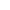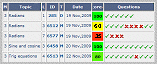Go to contentA challenge

In this magic square the four numbers in each row, column and diagonal add up to the same total. This total is called the Magic total.

The example used here is based on a 4x4 magic square from India, which can be seen in Khajuraho in the Parshvanath Jain temple. It dates from the 10th century.

It is referred to as the Chautisa Yantra, since each row, column, diagonal, 2x2 sub-square, the corners of each 3x3 and 4x4 square, and the sum of the middle two entries of the two outer columns and rows all add to the Magic total.

Because there are so many patterns, you have to find 10 missing numbers!